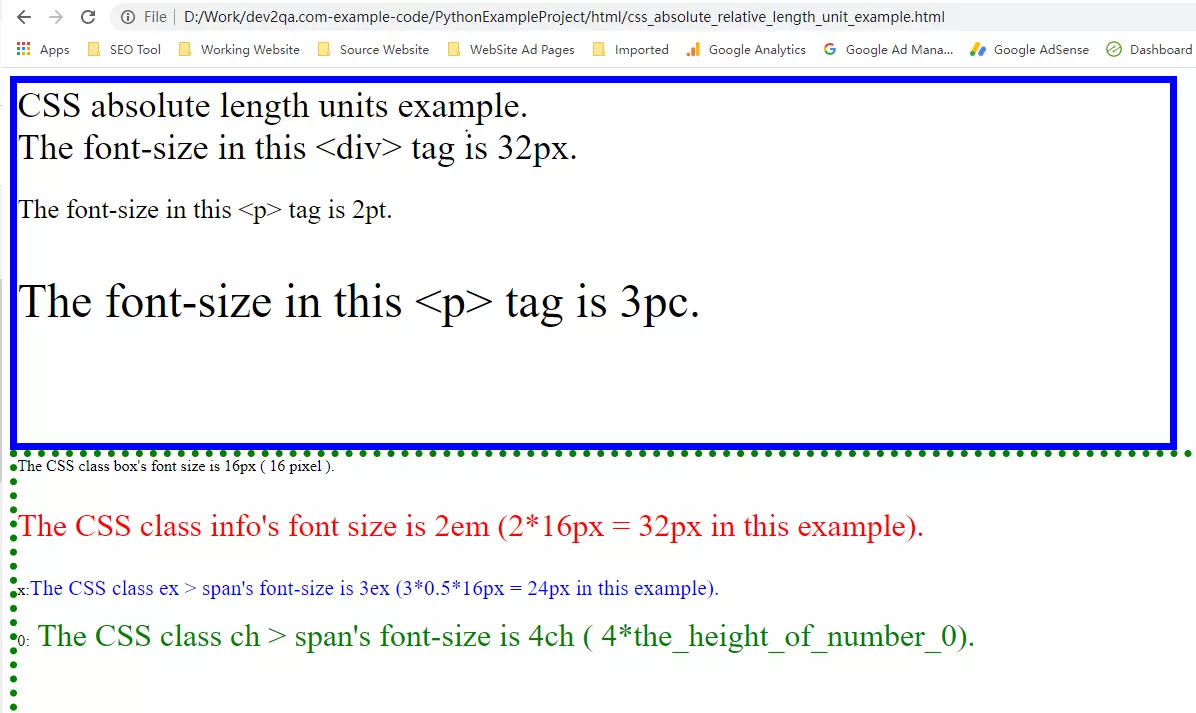# How To Use CSS Absolute And Relative Length Units Examples

CSS supports a variety of different length units, which can be divided into two groups according to different types: absolute length units (such as inches, centimeters, and points) and relative length units (such as percentage). This article will show you some examples of how to use CSS absolute and relative length units.

### 1. Absolute Length Units.

1. The absolute length unit represents a real physical size.
2. Its size is fixed and will not change due to the change in the size of other elements.
3. The following example lists the absolute length units supported in CSS。
4. cm: centimeter, div{font-size: 0.5cm;}.
5. in: inch (1in = 96px = 2.54cm), span{font-size: 1in;}.
6. mm: millimeter, p{font-size: 5mm;}.
7. pc: pica, 1pc = 12pt, p{font-size: 6pc;}.
8. pt: point, 1pt = 1/72in, p{font-size: 16pt;}.
9. px: pixel, 1px = 1/96in, p{font-size: 16px;}.

### 2. Relative Length Units.

1. %: percentage relative to parent element width or font size, p{width: 95%}.
2. ch: relative to the height of the number 0 in the font used, if the height of 0 cannot be determined, use 0.5em, p{line-height: 3ch}.
3. em: relative to the value of its own font-size attribute, if it is not set, it inherits the value of the font-size attribute of the parent element. 1em equals the value of the font-size attribute, for example, if the value of font-size is 16px, 1em equals 16px and 2em equals to 32px also, div{line-height:2em;}.
4. ex: relative to the height of the small written English letter x in the font used, if the height of x cannot be determined, use 0.5em instead, p{font-size: 1ex;}.
5. rem: relative to the size of the font-size attribute of the root element <HTML>, for example, if the font-size of the root element is 100px, then 1.2rem is equivalent to 120px, p{font-size: 1.2rem;}.
6. vh: relative to the height of the browser window, 1vh = 1% of the window height, p{font-size: 5vh;}.
7. vw: relative to the width of the browser window, 1vw = 1% of the window width, p{font-size: 5vw;}.
8. vmax: the larger value between vh and vw, p{font-size: 5vmax;}.
9. vmin: the smaller value between vh and vw, p{font-size: 5vmin;}.

### 3. CSS Absolute & Relative Length Unit Examples.

1. The below Html source code shows some examples of how to use CSS absolute & relative length units.
2. The Html file name is css_absolute_relative_length_unit_example.html, below is the file source code.
```<!DOCTYPE html>
<meta charset="ISO-8859-1">
<title>CSS Absolute & Relative Length Unit Examples</title>
<style>

/* below define CSS class use absolute length units. */

.absolute_box{
width: 12in;
height: 9.5cm;
border: 2mm solid blue;
font-size: 36px;
}

/* define the CSS class pt. */
.pt{
font-size: 20pt;
}

/* define the CSS class pc. */
.pc{
font-size: 3pc;
}

/* below define the CSS class use the relative lenght units. */

/* define the CSS box class styles. */
.box{
width: 90vw;/* define the width is 90% of the browser window width. */
height: 88vh; /* define the height is 88% of the browser window height. */
border: 1ex dotted green; /* define the border width is 1ex, the border style is dotted, the border color is green.  */
font-size: 16px; /* define the text font-size is 16px. */
}

/* define the CSS info class styles. */
.info{
font-size: 2em; /* 2em = 2*16px(the parent element font-size in this example ) = 32px. */
color: red
}

/* define the CSS class ex > span html element's style. */
.ex > span{
font-size: 3ex; /* 3ex = 3 * the_height_of_smaller_x or 3*0.5em ( 3*0.5*16px ). */
color: blue
}

/* define the CSS class ch > div html element's style. '*/
.ch > span{
font-size: 4ch; /* 4ch = 4*the_height_of_number_0 or 4*0.5em (4*0.5*16px) */
color:green
}

</style>

<body>

<div class="absolute_box">
CSS absolute length units example.<br/>
The font-size in this &lt;div&gt; tag is 32px.
<p class="pt">The font-size in this &lt;p&gt; tag is 2pt.</p>
<p class="pc">The font-size in this &lt;p&gt; tag is 3pc.</p>
</div>

<div class="box">
The CSS class box's font size is 16px ( 16 pixel ).
<p class="info">The CSS class info's font size is 2em (2*16px = 32px in this example).</p>
<p class="ex">
x:<span>The CSS class ex > span's font-size is 3ex (3*0.5*16px = 24px in this example).</span>
</p>
<p class="ch">
0:<span> The CSS class ch > span's font-size is 4ch ( 4*the_height_of_number_0).</span>
</p>
</div>
</body>
</html>```
3. When you browse the above Html file, you can see the page like below.This site uses Akismet to reduce spam. Learn how your comment data is processed.